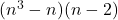How Cheenta works to ensure student success?
Explore the Back-Story

# Integer and Divisibility | B.Stat Objective | TOMATO 69Try this TOMATO problem from I.S.I. B.Stat Entrance Objective Problem based on Integer and Divisibility.

## Integer and Divisibility (B.Stat Objective problems)

Every integer of formfor n=3,4,..... is

• divisible by 12 but not always divisible by 24
• divisible by 6 but not always divisible by 12
• divisible by 24 but not always divisible by 48
• divisible by 9

### Key Concepts

Logic

Integers

Divisibility

Answer: divisible by 6 but not always divisible by 12

B.Stat Objective Question 69

Challenges and Thrills of Pre-College Mathematics by University Press

## Try with Hints(n-1)n(n+1) is divisible by 3 and any two consecutive integers is divisible by 2 gcd(2,3)=1

then 6|(n-1)n(n+1) and minimum (n-2)=1 for n=3,4,.... thendivisible by 6 but not always divisible by 12.

## Subscribe to Cheenta at Youtube

Try this TOMATO problem from I.S.I. B.Stat Entrance Objective Problem based on Integer and Divisibility.

## Integer and Divisibility (B.Stat Objective problems)

Every integer of formfor n=3,4,..... is

• divisible by 12 but not always divisible by 24
• divisible by 6 but not always divisible by 12
• divisible by 24 but not always divisible by 48
• divisible by 9

### Key Concepts

Logic

Integers

Divisibility

Answer: divisible by 6 but not always divisible by 12

B.Stat Objective Question 69

Challenges and Thrills of Pre-College Mathematics by University Press

## Try with Hints(n-1)n(n+1) is divisible by 3 and any two consecutive integers is divisible by 2 gcd(2,3)=1

then 6|(n-1)n(n+1) and minimum (n-2)=1 for n=3,4,.... thendivisible by 6 but not always divisible by 12.

## Subscribe to Cheenta at Youtube

This site uses Akismet to reduce spam. Learn how your comment data is processed.

### Knowledge Partner